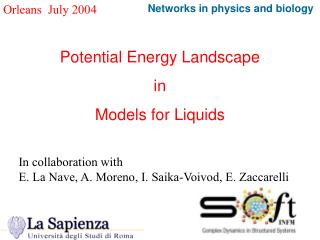DownloadDownload PresentationOrleans July 2004

# Orleans July 2004

Download Presentation## Orleans July 2004

- - - - - - - - - - - - - - - - - - - - - - - - - - - E N D - - - - - - - - - - - - - - - - - - - - - - - - - - -
##### Presentation Transcript

1. Orleans July 2004 Networks in physics and biology Potential Energy Landscape in Models for Liquids In collaboration with E. La Nave, A. Moreno, I. Saika-Voivod, E. Zaccarelli

2. A 3-slides preamble: Thermodynamics and Dynamics Review of thermodynamic formalism in the PEL approach Potential Energy Landscapes in Fragile and Strong (Network-Forming) liquids. Outline Outline

3. Strong and Fragile liquids Dynamics A slowing down that cover more than 15 order of magnitudes P.G. Debenedetti, and F.H. Stillinger, Nature 410, 259 (2001). 1

4. A Decrease in Configurational Entropy: Thermodynamics Why do we care Thermodyanmics Is the excess entropy vanishing at a finite T ? 1

5. The basic idea: Separation of time scales f(t) glass liquid f(t) van Megen and S.M. Underwood Phys. Rev. Lett. 70, 2766 (1993) Glass Supercooled Liquid log(t)

6. Potential Energy Landscape, a 3N dimensional surface Statistical description of the number, depth and shape of the PEL basins e IS P IS w The PEL does not depend on TThe exploration of the PEL depends on T

7. Z(T)= S Zi(T) allbasins i fbasin i(T)= -kBT ln[Zi(T)] fbasin(eIS,T)= eIS+ kBTSln [hwj(eIS)/kBT] + fanharmonic(eIS, T) normal modes j

8. Thermodynamics in the IS formalism Stillinger-Weber F(T)=-kBT ln[W(<eIS>)]+fbasin(<eIS>,T) with Basin depth and shape fbasin(eIS,T)= eIS+fvib(eIS,T) and Number of explored basins Sconf(T)=kBln[W(<eIS>)]

9. Distribution of local minima (eIS) Configuration Space + Real Space Vibrations (evib) rN evib eIS

10. <eIS>(T) (steepest descent minimization) fbasin(eIS,T) (harmonic and anharmonic contributions) F(T) (thermodynamic integration from ideal gas) From simulations….. F(T)=-kBT ln[W(<eIS>)]+fbasin(<eIS>,T) E. La Nave et al., Numerical Evaluation of the Statistical Properties of a Potential Energy Landscape, J. Phys.: Condens. Matter 15, S1085 (2003).

11. Fragile Liquids: The Random Energy Model for eIS Hypothesis: e-(eIS -E0)2/2s 2 W(eIS)deIS=eaN -----------------deIS 2ps2 S ln[wi(eIS)]=a+b eIS Predictions: <eIS(T)>=E0-bs 2 - s 2/kT Sconf(T)=aN-(<eIS (T)>-E0)2/2s 2

12. T-dependence of <eIS> SPC/E LW-OTP T-1 dependence observed in the studied T-range Support for the Gaussian Approximation

13. BMLJ Sconf BMLJ Configurational Entropy

14. Landscape Equation of State P=-∂F/∂V|T F(V,T)=-TSconf(T,V)+<eIS(T,V)>+fvib(T,V) In Gaussian (and harmonic) approximation P(T,V)=Pconst(V)+PT(V) T + P1/T(V)/T Pconst(V)= - d/dV [E0-bs2] PT(V) =R d/dV [a-a-bE0+b2s2/2] P1/T(V) = d/dV [s2/2R]

15. Non Gaussian Behaviour in BKS silica

16. Sconf Silica Non-Gaussian Behavior in SiO2 Eis e S conf for silica… Esempio di forte Non gaussian silica

17. Density minimum and CV maximum in ST2 water Density Minima P.Poole inflection in energy inflection = CV max

18. ST2 2650 punti Isochores of liquid ST2 water • HDL LDL ?

19. Maximum Valency Maximum Valency Model (Speedy-Debenedetti) V(r ) SW if # of bonded particles <= Nmax HS if # of bonded particles > Nmax r A minimal model for network forming liquids The IS configurations coincide with the bonding pattern !!!

20. It is possible to calculate exactly the basin free energy ! Basin Free energy

21. Ground State Energy Known ! It is possible to equilibrate at low T ! Energy per Particle

22. Viscosity and Diffusivity: Arrhenius

23. Configurational Entropy

24. Fragile Liquids Gaussian Energy Landscape Finite TK, Sconf(TK)=0 Strong Liquids: “Bond Defect” landscape (binomial) A “quantized” bottom of the landscape ! Degenerate Ground State Sconf(T=0) different from zero ! Suggestions for further studies…..

25. Acknowledgements We acknowledge important discussions, comments, collaborations, criticisms from… A. Angell, P. Debenedetti, T. Keyes, A. Heuer, G. Ruocco , S. Sastry, R. Speedy … and their collaborators

26. Stoke-Einstein Relation

27. Citazioni goldstein, stillinger

28. Gaussian Distribution ? eIS=SeiIS E0=<eNIS>=Ne1IS s2= s2N=N s21

29. Diffusivity

30. Phase Diagram NMAX-modifiedPhase Diagram

31. The V-dependence of a, s2, E0 e-(eIS -E0)2/2s 2 W(eIS)deIS=eaN -----------------deIS 2ps2

32. SPC/E P(T,V)=Pconst(V)+PT(V) T + P1/T(V)/T FS, E. La Nave, and P. Tartaglia, PRL. 91, 155701 (2003)

33. D ST2 Isobars of diffusion coefficient for ST2 water

34. Adam-Gibbs Plot

35. Basin Free Energy kBTSln [hwj(eIS)/kBT] LW-OTP SPC/E S ln[wi(eIS)]=a+b eIS …if b=0 …..

36. BKS Silica Eis nel tempo